## Sunday, November 20, 2011

### CALCULATION OF GSM

Learn Apparel Merchandising, Learn Fashion design.
Dear All,

GSM means the weight in gram per square meter of fabric.GSM is a very important parameter for specified a certain quality of knitted fabric. The production of knitted fabric is calculated in weight. The GSM cutter is very popular and easy usable GSM testing instrument used in most knitted factory. But the construction of this cutter is very simple. It is circular disk of 100 square cm area with sharp blade attached to its edge. So 100 square cm of fabric can easily cut by it and weighted at the electric balance to get GSM reading.

There are two formulas for calculating the GSM of a knitted fabric

First formula is as under:

Course per inch X Stitch length X 39.37 X 39.37 X Tex
GSM =    ----------------------------------------------------------------------
1000 X 1000

aNd

WPI X CPI X SL(mm)
GSM =    ----------------------------- X 0.9155
Count(Ne)

CALCULATION OF WALES PER INCH (WPI):

We calculated the number of Wales in 10 inch fabric unraveling the yarn. Then we divide the no of total Wales by 10 inch to getting the Wales per inch.

CALCULATION OF COURSE PER INCH (CPI):

We calculated the number of course in five inch with the help of counting glass and needle. Then we divide the total no of course by five inch to getting the course per inch.

MEASUREMENT OF STITCH LENGTH (SL mm):

Stitch length is theoretically a single length of yarn which includes one needle loop and adjacent needle loops on either side of it. Loop exits in course in course length and it is that which influence fabric dimension and other properties including weight.

In order to determine the stitch length, we count 100 no Wales or stitch and count its length by hanging the yarn on the stitch counter. The reading is found in mm unit.

MEASUREMENT OF YARN COUNT:

We have fallowed a different way to find out the count of the yarn. At first we unravel a considerable no yarn from the fabric. Then we measured the total length of the yarn and measured the weight of that no of yarn. From these weights, we find out the count of the yarn.

The equation we followed is as follow:

N x L x 453.6
Count =   ----------------
Wt x 36 x 840
Where,

N   = number of yarn in bundle.
L    = length of yarn.
Wt  = weight of yarn.

Another formula is the following:

Ks x Tex
GSM =   ---------------------
Stitch length (mm)

Where,
Ks is a constant. Its value is different for different fabric structure and fabric type. Ks is calculated and estimated as below:

GSM x Stitch length
Ks =     -----------------------------
Tex

(Collected & Edited)

Thanks & best regards
Bipul

1.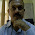good calculations...

what is dying p2 p3 p4

2.3.i want to know that relation between gsm & stitch length sacs as for 160 gsm s/j stitch length needed 2.6 for white & 2.65 for average colour here my question is how will we understand for 160 gsm s/l 2.6 or 2.65?
can you ans me?
shaharia3761@yahoo.com
01721903761

1.may we know what is ks?

4.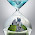Course per inch X Stitch length X 39.37 X 39.37 X Tex
here, what is TEx ?

thanks .......

5.Course per inch X Stitch length X 39.37 X 39.37 X Tex

what is Tex ?

Thanks

6.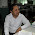How will get KG to yds - do you have formula ?

such as 1 kgs s/jsy , 160gs = how many yds will be there ??

1.Dear Kawser,

717* kg/ gsm= yards
1 kg 160 gsm = 4.48 yard

2.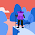Dear Ziyaur,

Best Regards
Enamul Haque Bipul

3.But Enamul and Ziyaur... is Yard is not depend on fabric width... Like if have 44 width fabric is different yard in 1 KG and 62 width of fabric is different yard in 1 KG even both weight will be 160 G/SM...

4.Enamul and ziyur... i think yard should be very depends on fabric width...
like 160 gsm .. yard will be different in 44" width and 62" width of fabric... pls. elaborate

7.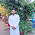vaiya ...my question is same fabric length 10m and width 68 inch gsm 200 how will be the kg of this fabric

8.SEAR KAWSAR FARUQUE
LENGTH IN YARDS= WT IN KGS *43055.2/WIDTH IN INCHES *2*GSM

1.Will you please explain it with some example..

Thanks,
Richa

2.Will you please explain the same with some example?

9.Example : i have 30's NE ( actual count 28.5) 100% cotton, Required gsm 150 to 155 , Machine GG 24 and 28gg, what stich length should i keep, how to calculate.

10.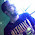I am new in garments sector . CPI*SL*39.37*39.97.Tex....Here what is Tex .Plz tell me how i get tex. Its Very rich site. Thank u Bipul sir

11.CPI*SL*39.37*39.37*Tex......here what is tex & how i get tex.....I hope u will give me the ans .Bipul Sir.

12.CPI*SL*39.37*39.97*Tex here what is tex & hw i get tex

1.Tex is the weight in grams of 1000 metres yarn.

2.Tex is the wt. in grams of 1000 metres yarn. Tex= (W*l/L)

3.Tex is the weight in grams of 1000 metres yarn.

13.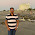Dear Sir,

14.How many GSM of 10cm X 10cm = 2.156gm?
Please let me know over cell number 01711125694.

15.How many GSM of interlining weight 10cm X 10cm = 2.156gm?

16.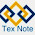Thanks for such a useful information. Really it is very helpful............
17.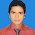18.19.20.